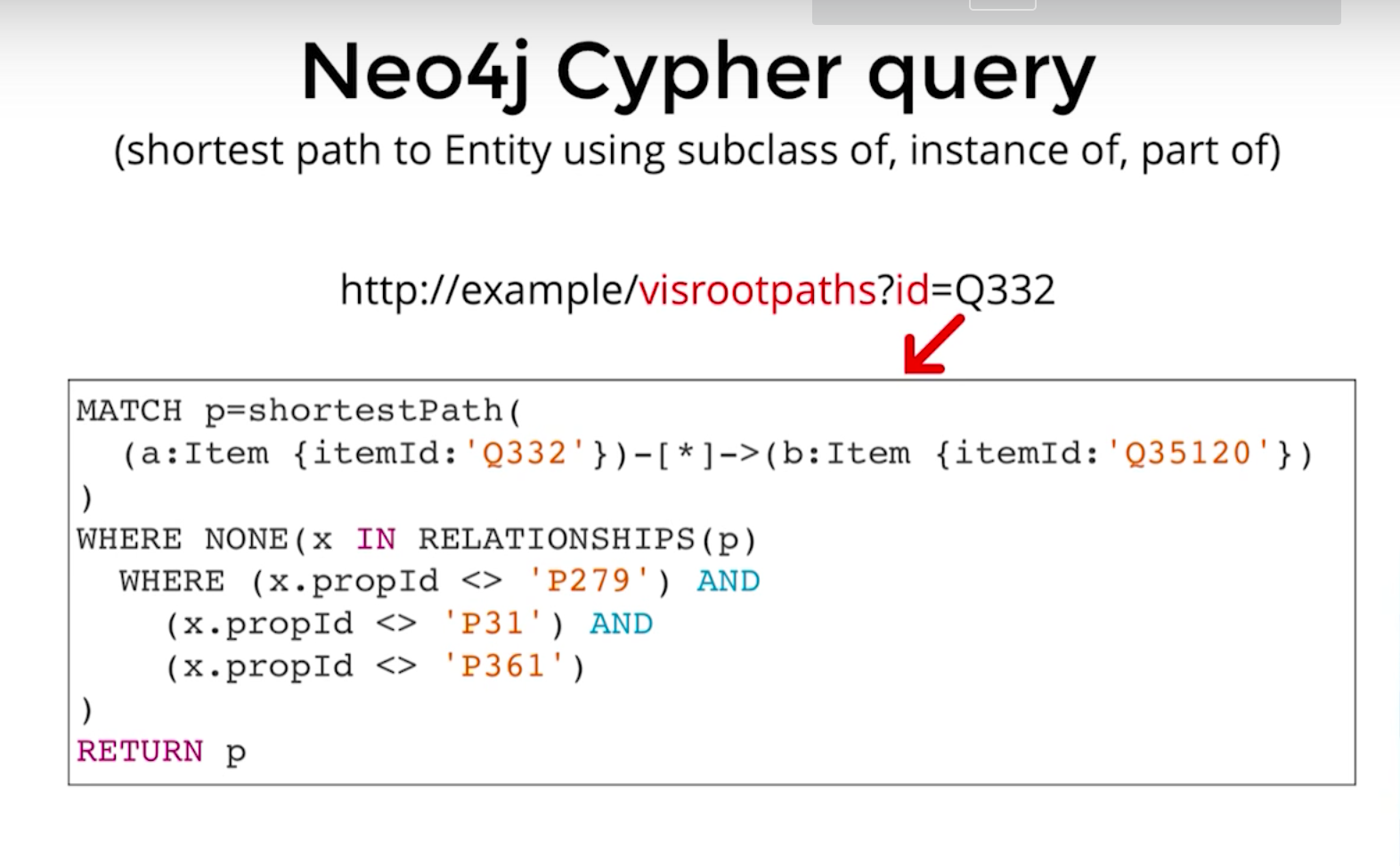# 03. Neo4j Cypher QueryExample of Neo4j ypher query

We are aware of SQL “Structured Query Language“ which we usually use in SQL databases. Here we are using CQL “Cypher Query Language“.

Neo4j is based on node and relations. Node can be considered as tables in SQL and relations can be considered as foreign keys in there.

What is CQL?

1. Is a query language for Neo4j Graph Database.
2. Is a declarative pattern-matching language.
3. Follows SQL like syntax.
4. The syntax is very simple and in human-readable format.
5. Neo4j CQL has commands to perform database operations.
6. Neo4j CQL supports many clauses such as WHERE, ORDER BY, etc., to write very complex queries in an easy manner.
7. Neo4j CQL supports some functions such as String, Aggregation. In addition to them, it also supports some Relationship Functions.

Lets understand pattern of CQL. After understanding pattern then it will be just matter of practice.

`MATCH (n:Person)-[:KNOWS]->(m:Person) WHERE n.name = 'Alice' RETURN m`
1. MATCH (n: Person) where n.name= ‘Alice’ it will find a Person with name ‘Alice’.
2. -[:KNOWS] : it signifies the relation between two nodes or entities. We can keep some properties with relations also that time we have to filter it out with name then we can write like [r : KNOWS]. Here r is relation alias and we can use “r.date_of_last_meet” kind of property in where clause.
3. -[:RELATION]-> : here we can see
4. “-[]-> “ : arrows . which signifies directed relation. Here in our case direction from Alice to other person.
5. “(a)<-[]-(b)“ : which states that relation from b to a.
6. “(a)-[]-(b)“ : if we want to keep relation bidirectional then use this.
7. RUTURN m — is just returning data fetched from database.
8. WHERE n.name = ‘Alice’ — Here we have used WHERE clause which we are already aware of in SQL also.

There are multiple clauses we can use like we have used in above query. Here are few:-

Read More..

All blogs..

https://param105.medium.com/

Software Engineer. (Mobile and Web)